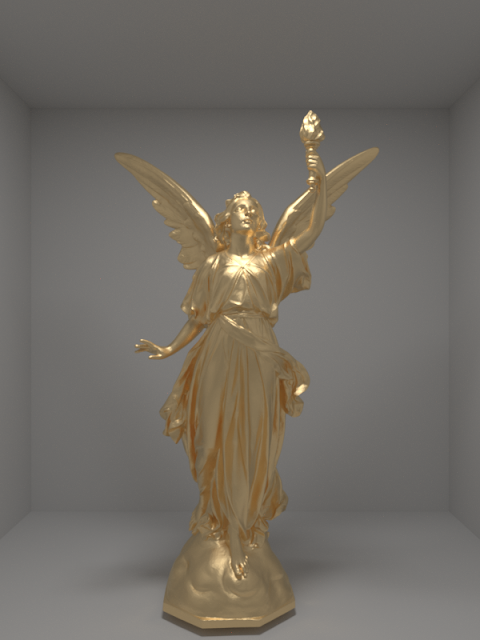### Microfacet Theory: Reflection From and Transmission Through Rough Surfaces

A Reflectance Model for Computer Graphics[CT82] introduced microfacet theory to the graphics. Since then, many improvements and simplifications over the model have been proposed. Since most of the terms that constitute the formula of the model are fixed, much of the research focused on different distribution function of normals and masking-shadowing functions.

Microfacet Models for Refraction through Rough Surfaces[WMLT07] extends the microfacet model for reflection from rough surfaces to refraction through rough surfaces. Although previous work can be found exactly on this subject, this paper is the first to validate the proposed model. It presents a reference for implementors by giving the sampling weights and steps to implement the model. Also, it proposes a new distribution function, GGX, which has longer tails than Beckmann distribution resulting in more realistic appearance for certain materials. The only drawback for this distribution is that it takes more to reduce variance since the tails are stronger.

Since this paper gives a brief overview on microfacet theory, you can start reading to dive in. However, if you feel confused, you can read relevant chapters(8, 14) of Physically Based Rendering and you can refer to the original paper [CT82].

Both GGX and Beckmann distributions are implemented in Glue. Also, microfacet reflection and microfacet scattering are separated. The latter includes both reflection and transmission. Both reflection and scattering are implemented as templates. Choosing between GGX and Beckmann distributions is handled in compile time. Thus, choice cannot be specified from the scene file. Also, there are two materials that make use of the microfacet theory in Glue. Metal instantiates a microfacet reflection and dielectric instantiates a microfacet scattering.

Relevant implementations are:Metal(Gold, GGX with alpha=0.15)Dielectric(IOR=1.5, GGX with alpha=0.001)Dielectric(IOR=1.5, GGX with alpha=0.027)Dielectric(IOR=1.5, GGX with alpha=0.15)